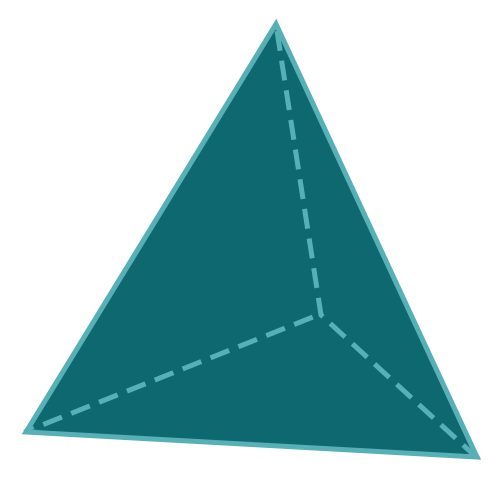Report a Problem
Suggestions

#Calculate The Surface Area of A Tetrahedron

Last updated: Saturday, April 29, 2023
Select a type of polyhedron below
Tetrahedron
Hexahedron
Octahedron
Dodecahedron
Icosahedron

The surface area of a tetrahedron is the total area of all its faces. A tetrahedron is a geometric shape with four triangular faces and four vertices. It is commonly used in the construction of pyramids and other architectural designs. The surface area of a tetrahedron can be calculated by finding the area of each face and adding them together.

In real life, tetrahedrons can be found in many constructions and objects. For example, the shape of a molecule of methane gas is a tetrahedron, as well as the pyramids of Egypt which were built using tetrahedral geometry. Additionally, tetrahedral structures are used in the design of space frames for building structures, as they are strong and can distribute weight evenly.

The formula for determining the surface area of a tetrahedron pyramid is defined as:
$$SA$$ $$=$$ $$\sqrt{3}$$ $$\cdot$$ $$a^2$$
$$SA$$: the surface area of the tetrahedron
$$a$$: the length of any side
The SI unit of surface area is: $$square \text{ } meter\text{ }(m^2)$$

## Find $$SA$$

Use this calculator to determine the surface area of a tetrahedron pyramid when the length of any side is given.
Hold & Drag
CLOSE
the length of any side
$$a$$
$$meter$$